Module 14 - Related Rates Introduction | Lesson 1 | Lesson 2 | Lesson 3 | Lesson 4 | Self-Test Lesson 14.4: A Moving Particle A final related-rate problem, which models a particle moving along a curve, is explored in this lesson. A particle is moving along the curve y = x3. At a certain instant, the particle is at the point (2, 8) and dx/dt = 5 ft/sec. How fast is the distance s from the particle to the origin changing at that instant?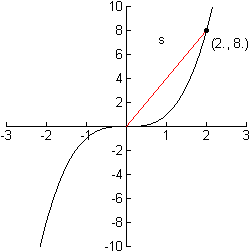Find the Distance Function s(t) Execute the NewProb command Define y(t)=(x(t))^3 Define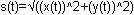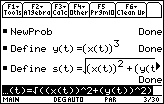Find dy/dt at the Point (2, 8) We know that dx/dt = 5 at this point. Thus, we need to compute dy/dt with the restrictions that x(t)=2, y(t)=8, and d(x(t),t)=5.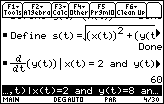So, dy/dt = 60 ft/sec at the point (2, 8). Answering the Question To find out how fast the distance s from the particle to the origin is changing at the specified instant, we need to compute ds/dt with the restrictions that x(t)=2, y(t)=8, dx/dt=5, and dy/dt=60.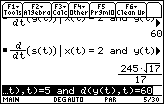Therefore, the particle is moving away from the origin at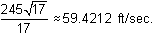< Back | Next > ©Copyright 2007 All rights reserved. | Trademarks | Privacy Policy | Link Policy